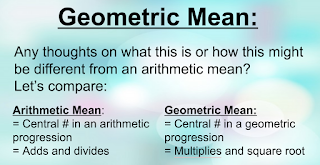Monday, January 29, 2018

Teaching Geometry - Geometric Mean #VNPS

I taught geometric mean years ago.  I did it with the different ratios within the triangle.  I did it with two numbers.  I wanted to have my kids discover it at the white boards and understand how to set up the ratios, so I started researched.

It was our first lesson as a new class with new students in the new term, so I wanted to get them used to my memes, so I showed this as my title of my slides and I got them up to the boards.In the process of researching the lesson, I learned two things:
1.) You can find the geometric mean of more than two numbers.  You just need to take that root.
2.) The geometric mean is the middle number in a geometric progression.

Here is the google slide lesson: Geometric Mean

I guess I just never really thought about #2 before but it made sense and I could relate it back to Algebra 1 when the students learned about geometric sequences.

When I introduce a new subject, I like to connect it to something they already know.  Naturally, that would be arithmetic mean.  We started with this:I asked what they thought Geometric Mean might do.  We worked our way to this:They did come up with the idea of multiplication.  I gave them two numbers and they had it.  They could calculate the geometric mean.  Then, I explained we would use proportions in similar triangles and use the geo mean to help us find other sides of the triangles.

Most of my whiteboarding (#VNPS) is oral, but I gave them this picture.

Here is my script for the board work.I asked them to classify it to orientate them with the picture.  I asked what "h" was and it took a bit but we got to the altitude.

I had them pull apart the three triangles so the right angle was in the left corner.  That was tough, but we got there.

Then, I asked them to write the ratio of short leg to longer leg.

I forgot to take a picture of that one, but here is a picture of student work for hypotenuse to short leg:And...voila...there it is staring at them - that diagonal geometric mean.  At least one group in the class had that aha moment and found the geometric mean.

We moved onto the second ratio and set it up.  And, a student taught me even more about the Geo Mean:
3.) The three geometric means are the three altitudes of the triangle!!!

I also thought of them as the altitude and the two legs, but yes, they are all altitudes.  How cool!

They set up the other ratios and found the geometric means within the proportions.  We came back to our desks to recap with notes and did a practice sheet.

I was happy with their work and their understanding.

1 comment:

1.Love the connection. Makes it more real. Thank you for sharing!